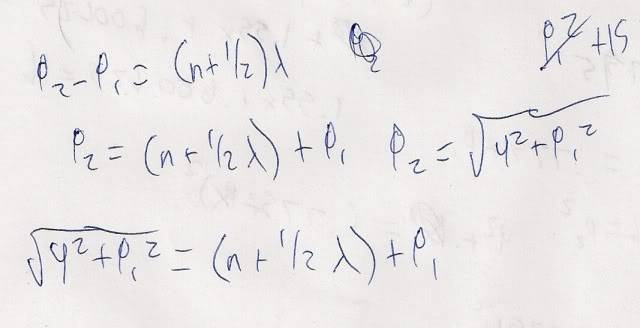# Destructive interference in a speaker value

## Homework Statement

Speaker 1 is positioned at the origin and speaker 2 is at the position (0, 4.00) meters. They emit identical sound waves of wavelength 1.55 m, in phase. If you stand on the x axis at (x, 0) meters, what is the smallest positive value for x for which you experience complete destructive interference?

A^2+ B^2 = C^2
P2-P1= (n+.5)λ
V= λf

## The Attempt at a Solution

My main question, though its possibly not where im going wrong, is would n have to equal 0 for it to be lowest destructive interference?

Excuse my poor handwriting. . .After plugging in all variables I came up with 9.94, which is incorrect. Is N supposed to equal 0 for this problem?

p1 is from the bottom speaker to the listener and p2 is the hypotenuse

The work you did seems to be on the right track, but perhaps use of different notation will clear things up. Since one speaker is located at the origin (0, 0) and the other speaker is located at (0, 4), we do not need the co-ordinates of the Point P1-P2 but rather its distance:

Since the Path Difference/ Wavelength = 0.5 (you had written n+0.5 but since we are looking for the smallest value, 0.5 will work), we can express the path difference as:
x units from the speaker at the origin
Sqrt(x^2 + 4^2) units from the speaker at (0, 4)

Thus: Path difference = Sqrt(x^2 + 4^2) - x
Wavelength = 1.55

The rest is for you to solve.

Hmm that gives me the answer I had already come up with which was incorrect, 9.935. Let me make sure I'm following you right

Path difference = Sqrt(x^2 + 4^2) - x

Here I'm plugging in wavelength/2, thus

.775 = Sqrt(x^2 + 4^2) - x
which brings me to
(x+.775)^2= x^2+ 4^2
which eventually goes to
1.55x-15.4=0
Thus x= 9.935. . . which is incorrect.

Ahh. I see my mistake. My mistake was assuming that the Path Difference/ Wavelength = 0.5. A value of 0.5 for the P.D./wavelength is not the smallest path difference. From a bit of trial and error, you will see that 2.5 (i.e. n=2, n+0.5 = 2,5) creates the smallest path difference with an answer of x=0.127.

I am sorry I have made such a basic error.